## A skater with a mass of 72 kg is traveling east at 5.8 m/s when he collides with another skater of mass 45 kg heading 60° south of west at 1

Question

A skater with a mass of 72 kg is traveling east at 5.8 m/s when he collides with another skater of mass 45 kg heading 60° south of west at 12 m/s. If they stay tangled together, what is their final velocity?

in progress 0
2 weeks 2021-09-05T14:24:01+00:00 1 Answers 0 views 0

1. The final velocity is 5.87 m/s

Explanation:

Given-

mass,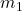= 72 kg

speed,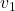= 5.8 m/s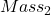,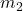= 45 kg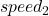,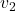= 12 m/s

Θ = 60°

Final velocity, v = ?

Applying the conservation of momentum:X+X= (+) v

72 X 5.8 + 45 X 12 X cos 60° = (72 + 45) v

v = 417.6 + 540 X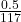v = 417.6 +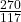v = 5.87 m/s

The final velocity is 5.87 m/s